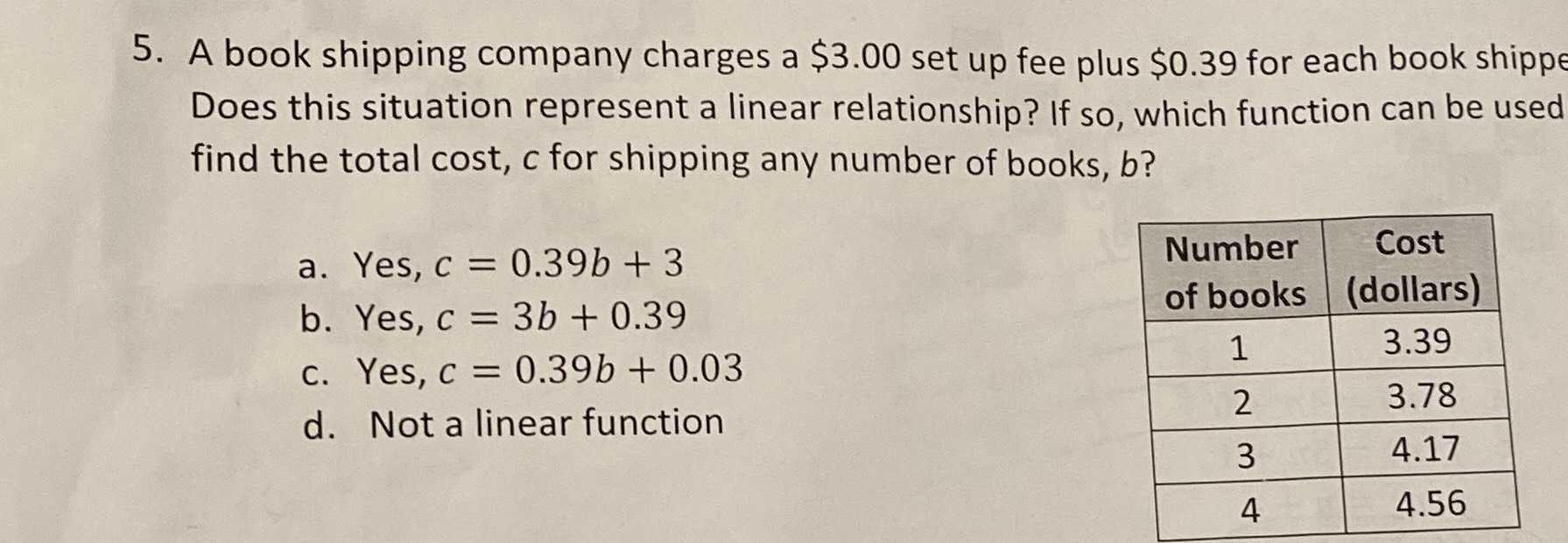### ¿Todavía tienes preguntas de matemáticas?

Pregunte a nuestros tutores expertos
Algebra
Pregunta5. A book shipping company charges a $$\ 3.00$$ set up fee plus $$\ 0.39$$ for each book shippe Does this situation represent a linear relationship? If so, which function can be used find the total cost, $$c$$ for shipping any number of books, $$b$$ ?

a. Yes, $$c = 0.39 b + 3$$

b. Yes, $$c = 3 b + 0.39$$

c. Yes, $$c = 0.39 b + 0.03$$

d. Not a linear function